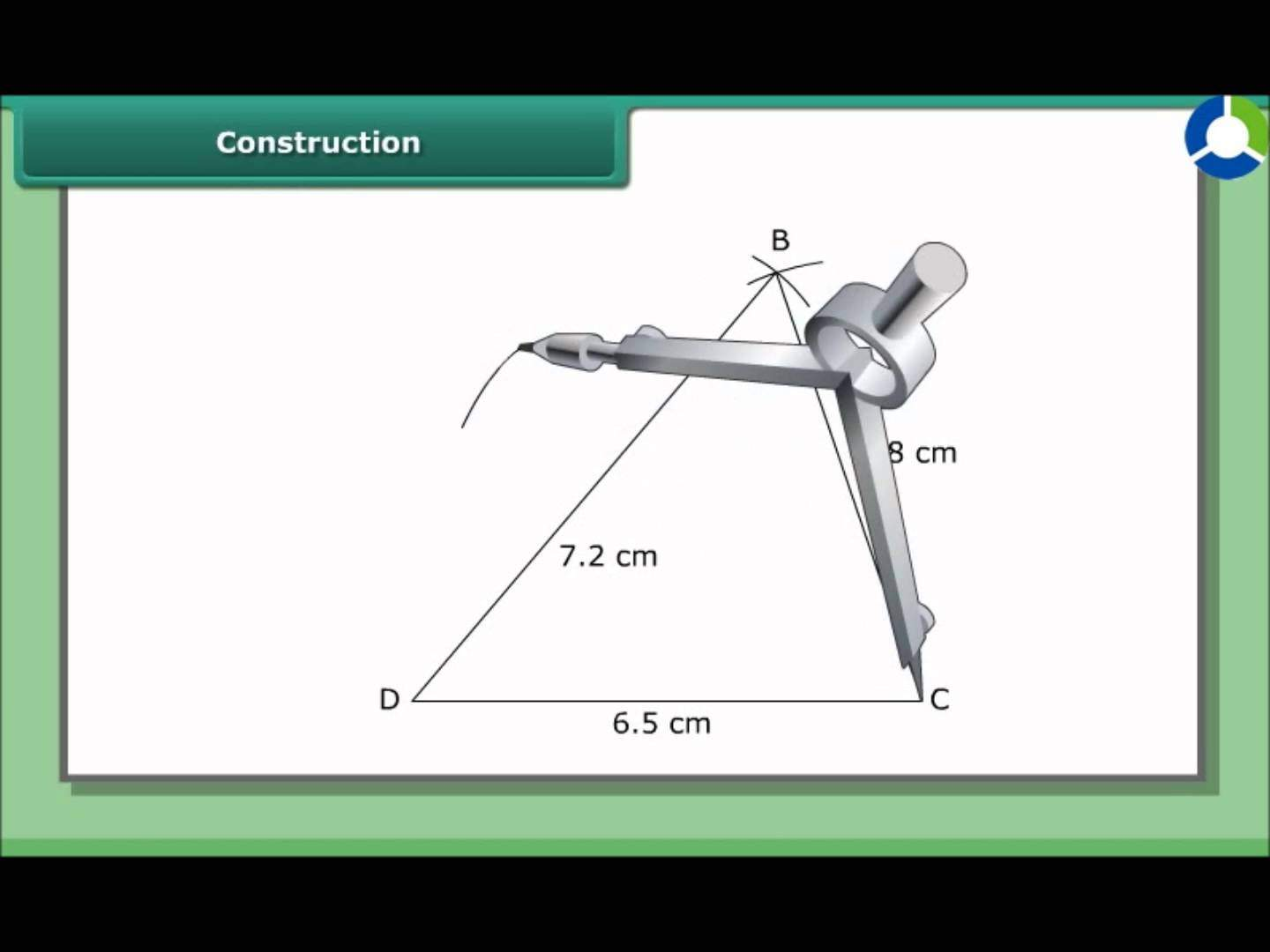# Practical Geometry worksheet for class 8## myCBSEguide App

CBSE, NCERT, JEE Main, NEET-UG, NDA, Exam Papers, Question Bank, NCERT Solutions, Exemplars, Revision Notes, Free Videos, MCQ Tests & more.

## Practical Geometry worksheet for class 8 Important Topics

• Introduction
• Some Special Cases## Some important Facts about Practical Geometry worksheet for class 8

1. Five measurements can determine a quadrilateral uniquely.
2. A quadrilateral can be constructed uniquely if the lengths of its four sides and a diagonal is given.
3. A quadrilateral can be constructed uniquely if its two diagonals and three sides are known.
4. A quadrilateral can be constructed uniquely if its two adjacent sides and three angles are known.
5. A quadrilateral can be constructed uniquely if its three sides and two included angles are given.

## NCERT Class 8 Mathematics Solved Worksheets

• Chapter 1: Rational Numbers
• Chapter 2: Linear Equations in One Variable
• Chapter 4: Practical Geometry
• Chapter 5: Data Handling
• Chapter 6: Squares and Square Roots
• Chapter 7: Cubes and Cube Roots
• Chapter 8: Comparing Quantities
• Chapter 9: Algebraic Expressions and Identities
• Chapter 10: Visualising Solid Shapes
• Chapter 11: Mensuration
• Chapter 12: Exponents and Powers
• Chapter 13: Direct and Inverse Proportions
• Chapter 14: Factorisation
• Chapter 15: Introduction to Graphs
• Chapter 16: Playing with Numbers

## CBSE Worksheets for Class 8 Mathematics in PDF

We shall learn how to construct a unique quadrilateral given the following measurements:

• When four sides and one diagonal are given.
• When two diagonals and three sides are given.
• When two adjacent sides and three angles are given.
• When three sides and two included angles are given.
• When other special properties are known.

## Draw the following

1. The square READ with RE = 5.1 cm.
2. A rhombus whose diagonals are 5.2 cm and 6.4 cm long.
3. A rectangle with adjacent sides of lengths 5 cm and 4 cm.
4. A parallelogram OKAY where OK = 5.5 cm and KA = 4.2 cm. Is it unique?

To download Printable worksheets for class 8 Mathematics and Science; do check myCBSEguide app or website. myCBSEguide provides sample papers with solution, test papers for chapter-wise practice, NCERT solutions, NCERT Exemplar solutions, quick revision notes for ready reference, CBSE guess papers and CBSE important question papers. Sample Paper all are made available through the best app for CBSE students and myCBSEguide website.